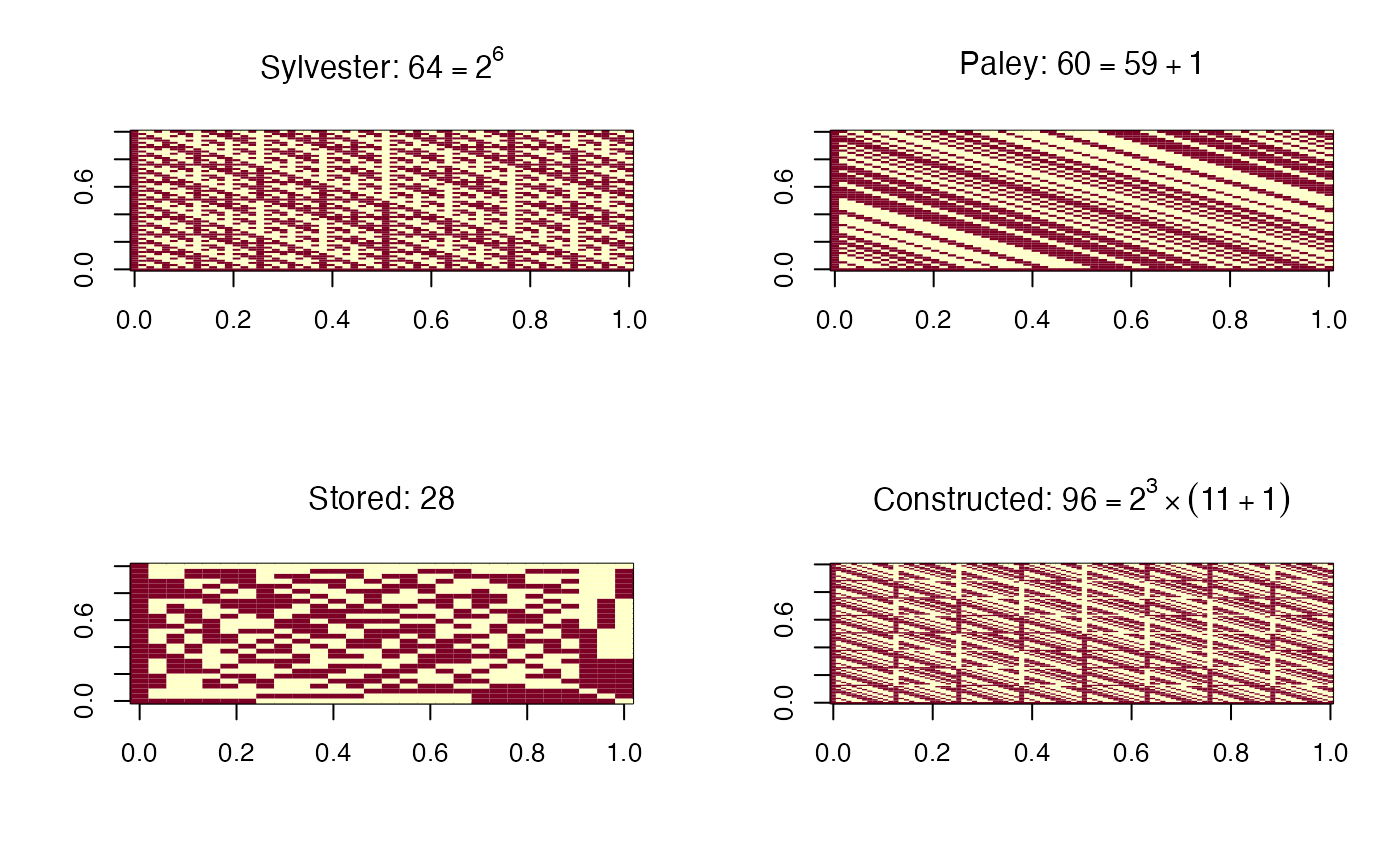Returns a Hadamard matrix of dimension larger than the argument.

hadamard(n)

## Arguments

n

lower bound for size

## Details

For most n the matrix comes from paley. The $$36\times 36$$ matrix is from Plackett and Burman (1946) and the $$28\times 28$$ is from Sloane's library of Hadamard matrices.

Matrices of dimension every multiple of 4 are thought to exist, but this function doesn't know about all of them, so it will sometimes return matrices that are larger than necessary. The excess is at most 4 for $$n<180$$ and at most 5% for $$n>100$$.

## Note

Strictly speaking, a Hadamard matrix has entries +1 and -1 rather than 1 and 0, so 2*hadamard(n)-1 is a Hadamard matrix

brrweights, paley

## Examples


par(mfrow=c(2,2))
## Sylvester-type
## Paley-type
## from NJ Sloane's library
## For n=90 we get 96 rather than the minimum possible size, 92.